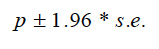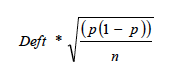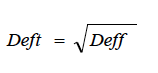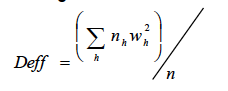Publication - Statistics

# Scottish Health and Care Experience Survey 2013/14 - Technical Report

Published: 17 Mar 2015
Part of:
Health and social care
ISBN:
9781785442070

Scottish Health and Care Experience Survey 2013/14. This is a postal survey which was sent to a random sample of patients who were registered with a GP in Scotland in October 2013. This report contains details of the survey design and development.

Scottish Health and Care Experience Survey 2013/14 - Technical Report
Annex D Calculation of confidence intervals

### Annex D Calculation of confidence intervals

8.1 The 95% Confidence Intervals for NHS Boards in Table 13 were calculated using the following formula:The s.e (standard error) was calculated as:Where:
p= point estimate (i.e. proportion who answered positively)
n= achieved sample size (i.e. number who answered the question).
And the design factor (Deft) was calculated as:Where Deff is the design effect and is calculated as:wh= weight given to each stratum h, where each GP practice was a stratum
n= achieved sample size (i.e. number who answered the question)
nh= achieved sample size in each stratum h.

 Example of calculation of confidence interval for NHS Ayrshire and Arran (Table 13) For NHS Ayrshire and Arran, where the result (proportion of patients rating the overall rating of care provided by GP Surgery as excellent or good) is equal to 86.79%, and the total sample size is 6,530. Then p = 0.8679, n = 6,530 deft= 1.10154 SE = deft*√(p(1-p))/n = 1.1054*√((0.8679(1-0.8679))/6,530 = 1.1054*0.00441 = 0.00486 95% Confidence Interval (CI) = 1.96 x SE CI = 1.96 x 0.00486 = 0.00953 Therefore the CI, expressed as a percentage (i.e. multiplied by 100) is ±0.95% The 95% confidence interval for NHS Ayrshire and Arran, where the proportion is 86.8%, is 85.8% - 87.7%.

### Contact

Email: Andrew Paterson